# Problem: A block lies on a plane raised an angle  from the horizontal. Three forces act upon the block:, the force of gravity; , the normal force; and , the force of friction. The coefficient of friction is large enough to prevent the block from sliding (Intro 1 figure).Which forces lie along the axes of the coordinate system b, in which the y-axis is vertical?

###### FREE Expert Solution

Fw points in the negative y-axis.

94% (405 ratings)###### Problem Details

A block lies on a plane raised an angle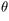from the horizontal. Three forces act upon the block: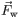, the force of gravity;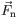, the normal force; and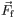, the force of friction. The coefficient of friction is large enough to prevent the block from sliding (Intro 1 figure).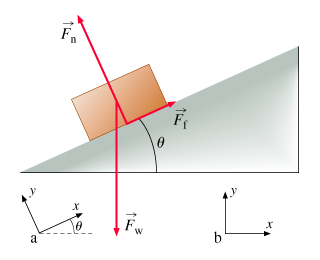Which forces lie along the axes of the coordinate system b, in which the y-axis is vertical?